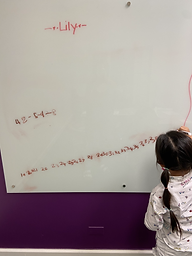## Ms. Joan

### Target 1​

###### Lesson Type:

Continuation

Number Operation

:

Computation

Subtract within 50.

###### 1:

Understand that the same process is utilized to add three or more whole numbers that is used to add only two numbers.

Kindergarden

###### Vocabulary:

Number line, Take a way

Activities:

• Students were given 3 or more number sentence to solve. They can use number line to find out the difference.
• Made their own number line on the board to answer the equation.### Home Exploration

###### Challenge Problem:

Subtract the following:

49-10-7-3 = ? (29)

30 - 11-7 = ? (12)

43-3-10-4 = ? (26)

###### Guiding Questions:
1. Start counting from the first jump from the biggest number.
2. Take away one at a time using the number line.## Absent Students:

### Target 2

:

###### 1:

Solve problems that have more than one operation, addition and subtraction.

###### 2:

Understand when solving a problem that has both addition and subtraction operations that solving starts on the left and continues to the right.

Kindergarden

###### Vocabulary:

Activities:

• Students were given set of number sentence with comnination of addition and subtraction using number line.
• Read the equation and figure what is the missing number.### Home Exploration

Find the ? in the given equation:

10+12+11-?= 20

Solve all the addition first then in the number line, find out how many jumps do you need to do to reach 20?

13

###### Guiding Questions:### Target 3

:

###### Vocabulary:

Activities:### Home Exploration